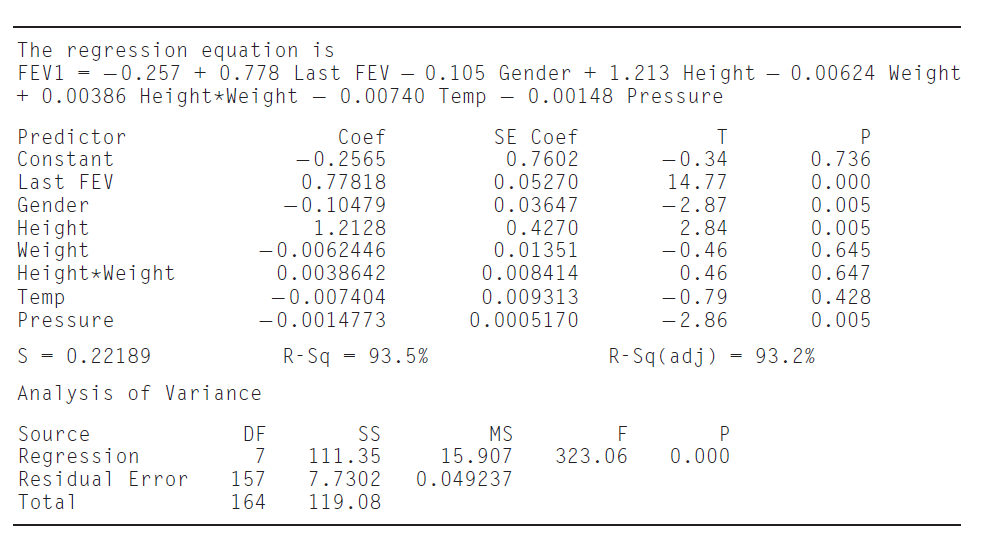×
Get Full Access to Statistics For Engineers And Scientists - 4 Edition - Chapter 8.3 - Problem 9e
Get Full Access to Statistics For Engineers And Scientists - 4 Edition - Chapter 8.3 - Problem 9e

×

# (Continues Exercise 7 in Section 8.1.) To try to improveISBN: 9780073401331 38

## Solution for problem 9E Chapter 8.3

Statistics for Engineers and Scientists | 4th Edition

• Textbook Solutions
• 2901 Step-by-step solutions solved by professors and subject experts
• Get 24/7 help from StudySoup virtual teaching assistantsStatistics for Engineers and Scientists | 4th Edition

4 5 1 255 Reviews
14
3
Problem 9E

(Continues Exercise 7 in Section 8.1.) To try to improve the prediction of FEV1, additional independent variables are included in the model. These new variables are Weight (in kg), the product (interaction) of Height and Weight, and the ambient temperature (in ◦C). The following MINITAB output presents results of fitting the model

FEV1 =β0 + β1 Last FEV1 + β2 Gender + β3 Height + β4 Weight + β5 Height · Weight + β6 Temperature + β7 Pressure + εa. The following MINITAB output, reproduced from Exercise 7 in Section 8.1, is for a reduced model in which Weight, Height · Weight, and Temp have been dropped. Compute the F statistic for testing the plausibility of the reduced model.b. How many degrees of freedom does the F statistic have?

c. Find the P-value for the F statistic. Is the reduced model plausible?

d. Someone claims that since each of the variables being dropped had large P-values, the reduced model must be plausible, and it was not necessary to perform an F test. Is this correct? Explain why or why not.

e. The total sum of squares is the same in both models, even though the independent variables are different. Is there a mistake? Explain.

Step-by-Step Solution:
Step 1 of 3

Chapter 2: Measurement 2.1 Significant Figures A. Accuracy and Precision 1) Accuracy: how close a measured value is to _____ the actual value__. 2) Precision: how close measured values are to _each other (average)_ B. Measured numbers • There will be some _uncertainty_ in where to round off a measured quantity based upon the precision of the measuring instrument. • The level of uncertainty is reported by using Significant Figures_ • The _more_ Significant Figures reported, the _more certain_ the measurement. C. Significant Figures (SF) 1 1) A number is Significant if it is: a) Not zero

Step 2 of 3

Step 3 of 3

##### ISBN: 9780073401331

Unlock Textbook Solution